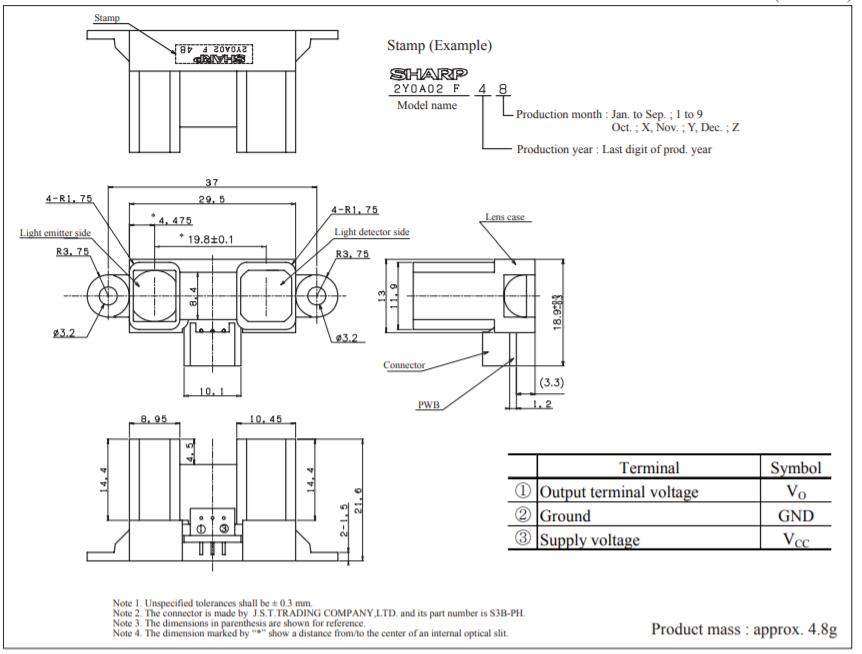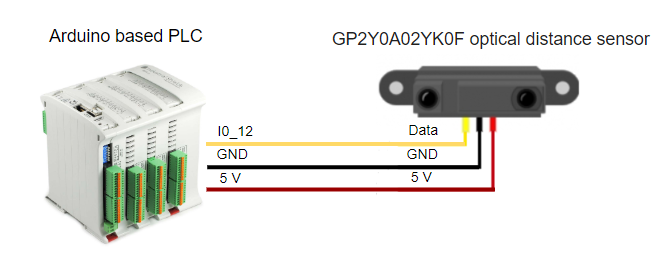# Measuring distances using an optical distance sensor

## Introduction

The Sharp GP2Y0A02YK0F is an optical distance sensor. It consists of an infrared LED together with a position detector device (PSD) and an integrated processor responsible for calculating the distance.

The sensor continuously scans the objects located in front of it and provides the output through an analog voltage reference, so we can use the Arduino analog inputs to read the value of the distance.

The measuring range is from 20 to 150 cm, maintaining a high degree of precision throughout the interval. The supply voltage is 4.5 to 5.5V and the current consumption is 33mA. The refresh interval between measurements is about 80ms.

The GP2Y0A02YK0F is a simple device to connect and use. However, it must be taken into account that it incorporates a JST (Japanese Solderless Terminal) connector for its connection, so we will have to use a connector of this type or directly solder the terminals on the board.

## Requirements

Ethernet PLC or 20 I/Os PLC:

GP2Y0A02YK0F optical distance sensor:

Industrial Shields boards:

##Characteristics Supply voltage (Vcc) (-0.3 to 7) V Output terminal voltage (Vo) (-0.3 to Vcc+ 0.3) V Operating temperature (Topr) (-10 to +60) ºC Storage temperature (Tstg) (-40 to +70) ºC Average supply current (Icc) typ. 30mA, max. 50mA Measuring distance range (ΔL) (20 to150) cm Output Voltage (V0) (mín. 0.25 ; typ. 0,4 ; max. 0,55) V

## Connections

GP2Y0A02YK - Arduino Based PLC wiring connections:

Pin 1 (Data)    -->  I0_12

Pin2 (Gnd) --> Gnd

Pin3 (5V) --> 5V

Final Connection:## Software

### As a test we will check the result monitored by the serial port checking the analog Value readed, the voltage received and finally the distance between the sensor and the object.

const long referenceMv = 5000;

void setup() {

Serial.begin(9600);

}

void loop() {

//lectura de la tensión

int mV = (val * referenceMv) / 1023;

int cm = getDistance(mV);

//mostrar valores por pantalla

Serial.print(mV);

Serial.print(",");

Serial.println(cm);

delay(1000);

}

//interpolación de la distancia a intervalos de 250mV

const int TABLE_ENTRIES = 12;

const int INTERVAL  = 250;

static int distance[TABLE_ENTRIES] = {150,140,130,100,60,50,40,35,30,25,20,15};

int getDistance(int mV) {

if (mV > INTERVAL * TABLE_ENTRIES - 1)      return distance[TABLE_ENTRIES - 1];

else {

int index = mV / INTERVAL;

float frac = (mV % 250) / (float)INTERVAL;

return distance[index] - ((distance[index] - distance[index + 1]) * frac);

}

}